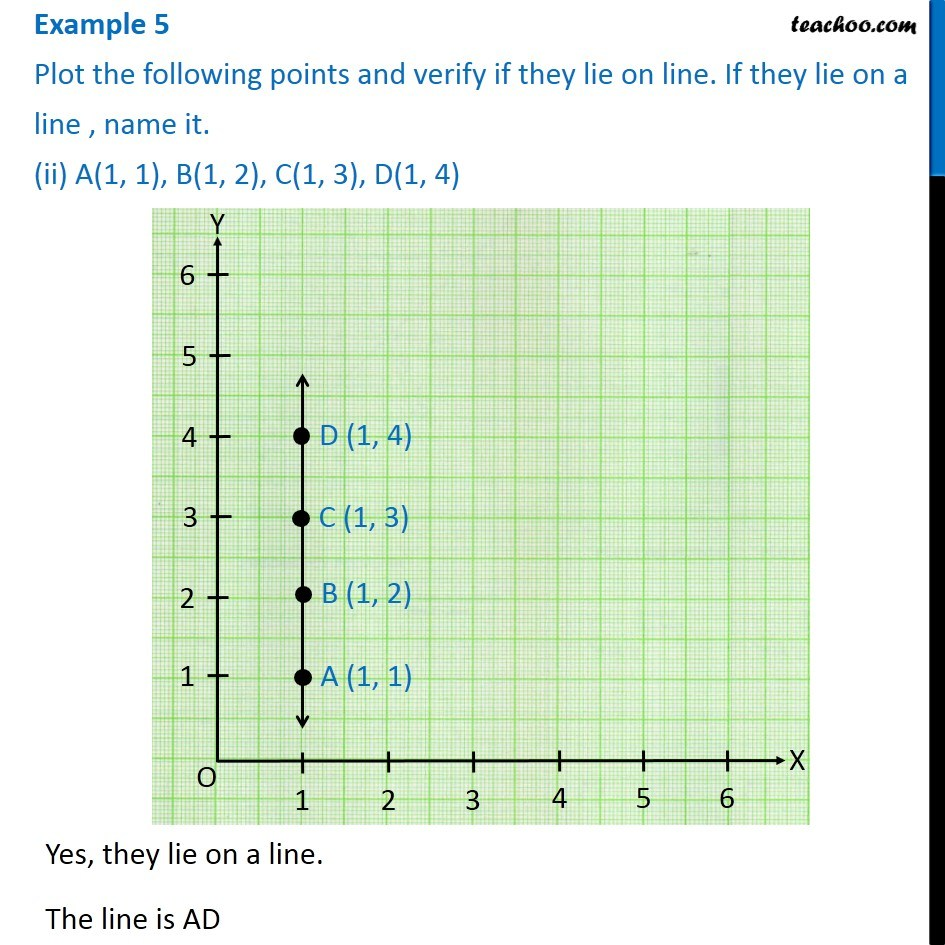1. Chapter 15 Class 8 Introduction to Graphs
2. Serial order wise
3. Examples

Transcript

Example 5 Plot the following points and verify if they lie on line. If they lie on a line , name it. (ii) A(1, 1), B(1, 2), C(1, 3), D(1, 4) Yes, they lie on a line. The line is AD